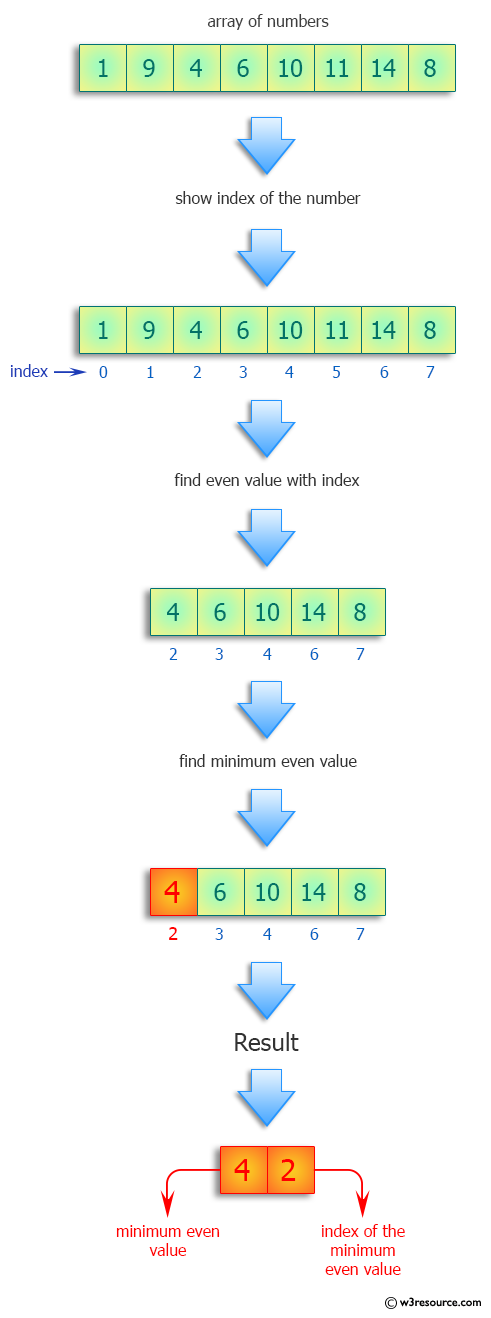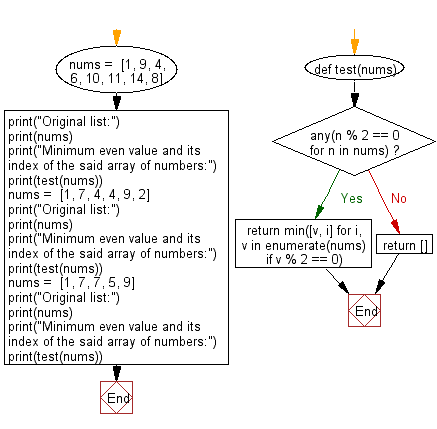﻿ Python: Find the minimum even value and its index from a given array of numbers - w3resource# Python: Find the minimum even value and its index from a given array of numbers

## Python Programming Puzzles: Exercise-46 with Solution

Given an array of numbers representing a branch on a binary tree, write a Python program to find the minimum even value and its index. In the case of a tie, return the smallest index. If there are no even numbers, the answer is [].

```Input:
[1, 9, 4, 6, 10, 11, 14, 8]
Output:
Minimum even value and its index of the said array of numbers:
[4, 2]
Input:
[1, 7, 4, 4, 9, 2]
Output:
Minimum even value and its index of the said array of numbers:
[2, 5]
Input:
[1, 7, 7, 5, 9]
Output:
Minimum even value and its index of the said array of numbers:
[]
```

Pictorial Presentation:Sample Solution:

Python Code:

``````#License: https://bit.ly/3oLErEI

def test(nums):
if any(n % 2 == 0 for n in nums):
return min([v, i] for i, v in enumerate(nums) if v % 2 == 0)
else:
return []

nums =  [1, 9, 4, 6, 10, 11, 14, 8]
print("Original list:")
print(nums)
print("Minimum even value and its index of the said array of numbers:")
print(test(nums))
nums =  [1, 7, 4, 4, 9, 2]
print("Original list:")
print(nums)
print("Minimum even value and its index of the said array of numbers:")
print(test(nums))
nums =  [1, 7, 7, 5, 9]
print("Original list:")
print(nums)
print("Minimum even value and its index of the said array of numbers:")
print(test(nums))
``````

Sample Output:

```Original list:
[1, 9, 4, 6, 10, 11, 14, 8]
Minimum even value and its index of the said array of numbers:
[4, 2]
Original list:
[1, 7, 4, 4, 9, 2]
Minimum even value and its index of the said array of numbers:
[2, 5]
Original list:
[1, 7, 7, 5, 9]
Minimum even value and its index of the said array of numbers:
[]
```

Flowchart:## Visualize Python code execution:

The following tool visualize what the computer is doing step-by-step as it executes the said program:

Python Code Editor :

Have another way to solve this solution? Contribute your code (and comments) through Disqus.

What is the difficulty level of this exercise?

Test your Programming skills with w3resource's quiz.

﻿

## Python: Tips of the Day

Clamps num within the inclusive range specified by the boundary values x and y:

Example:

```def tips_clamp_num(num,x,y):
return max(min(num, max(x, y)), min(x, y))
print(tips_clamp_num(2, 4, 6))
print(tips_clamp_num(1, -1, -6))
```

Output:

```4
-1
```